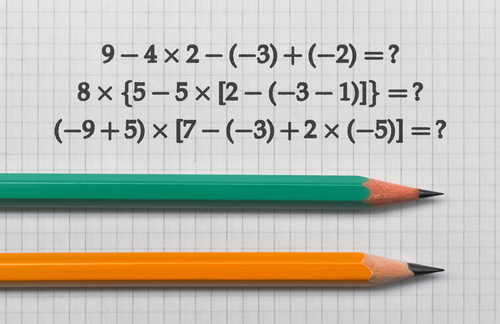# Negative Numbers used in Basic Algebra

Basic algebra students in Fullerton have been studying negative numbers for years. At first, negative numbers can seem difficult, even ominous. How can a number be less than zero? How can a person add, subtract, multiply, or divide an algebraic expression with negative numbers?But with the help of A Plus In Home algebra tutors in Fullerton, negative numbers in basic algebra can quickly become easy!

First off, what is a negative number?  In basic algebra (and all math), a negative number is less than zero.  Fullerton banks and financial experts use negative numbers all the time, and an Algebra tutor can give you real-life examples too.  One is debt.  If a student owes money or has a bank account balance of less than zero due to overspending, they are in the negative.  Another example is in very cold temperatures.  When it is below freezing outside, it is below zero degrees Celsius.  The temperature outside is negative.  Another way to explain negative numbers is with subtraction.  A student may have an “Ah-ha!” moment when their home algebra tutor explains that subtraction is the same as adding a negative number. Negative numbers can also be explained by using a number line.  In the picture above, everything to the left of zero is a negative number.

Basic algebra and pre-algebra students can have a home algebra tutor help them draw a number line when they get stuck on simple addition and subtraction problems with negative numbers.

A basic algebraic expression using negative numbers could look like the following: -3x + (-4) = 9 A tutor may suggest that the student will find it easier to write this as a subtraction problem: -3x – 4 = 9

From there, an effective home algebra tutor will show their algebra student how to add four to both sides to eliminate the negative number:

-3x – 4 + 4 = 9 + 4 -3x = 12 We have another negative number, the negative three.  However, the same steps as with a positive algebraic expression apply.  Divide by the same number on both sides, even if it is negative: -3x/-3 = 12/-3 X = 4 These are a few simple examples of negative numbers used in Algebra. If you or your student is having a hard time understanding Algebra,

A Plus In Home algebra tutor offers basic algebra tutoring to increase your understanding and improve your grades.

We have Home algebra tutors who will come to your home in Fullerton, Brea, Yorba Linda, and Anaheim providing tutoring in other subjects as well throughout Orange County.